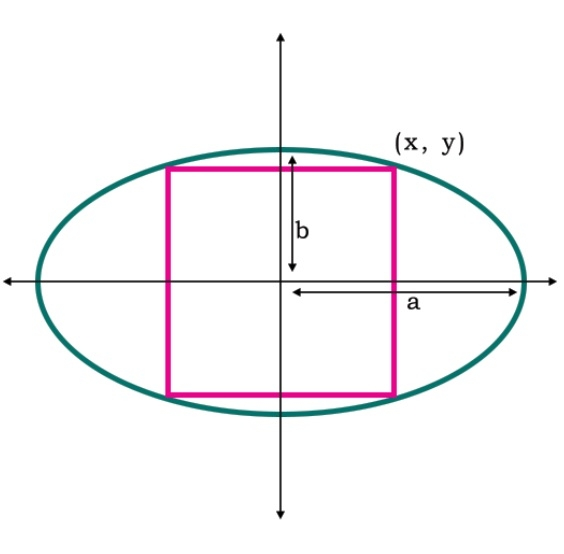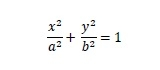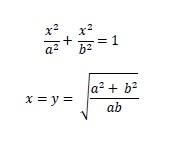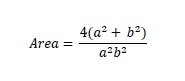# Area of the Largest square that can be inscribed in an ellipse in C++

Here we will see the area of largest square that can be inscribed in an ellipse. The square in ellipse will be like below −The area of ellipse is −Now, if x and y are same, thenSo area is −## Example

#include <iostream>
#include <cmath>
using namespace std;
float area(float a, float b) {
if (a < 0 || b < 0 ) //if values are is negative it is invalid
return -1;
float area = (4*(a*a + b*b)) / (a*a*b*b);
return area;
}
int main() {
float a = 4, b = 2;
cout << "Area : " << area(a, b);
}

## Output

Area : 1.25